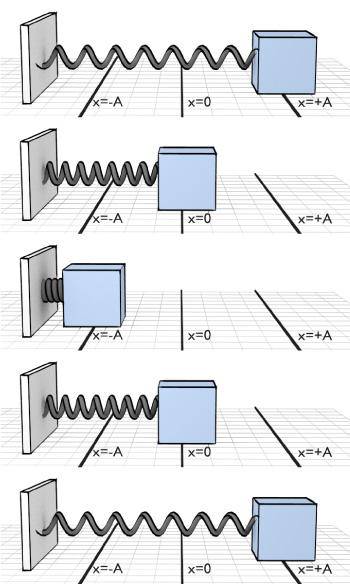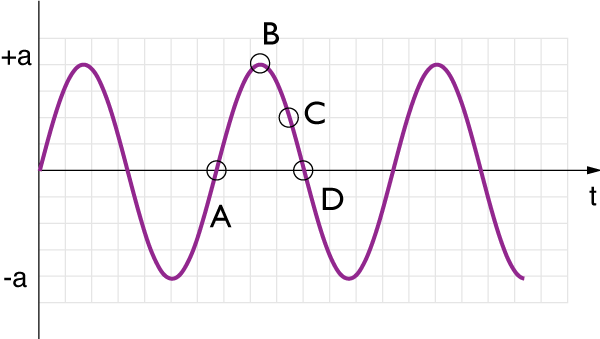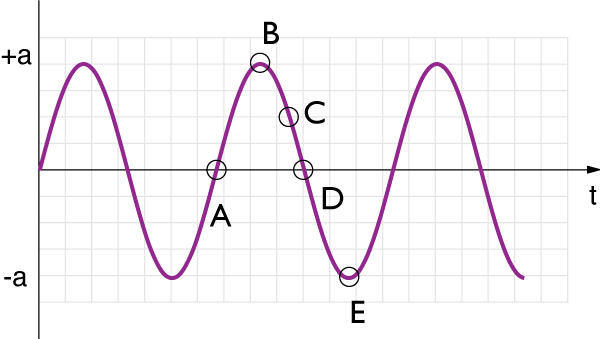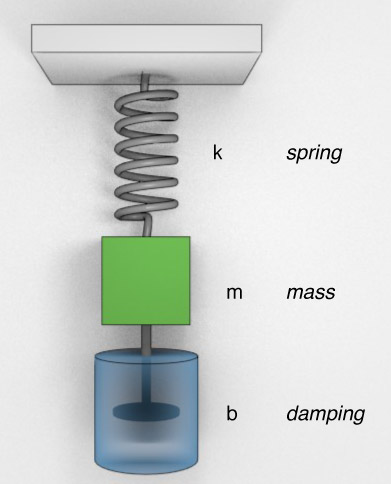# Oscillations

### Periodic Motion### Sinusoidal Motion

The simplest case is a sinusoidal motion. Here the graph has a sine or cosine shape.$$x(t) = \sin(t) \;\;\mbox{ or } \;\;x(t) = \cos(t)$$

This type of motion is called "simple harmonic motion"

### How do we get this kind of motion?

Simple harmonic motion will result from a restoring force, like Hooke’s Law: $$\bbox[10px ,border:3px solid red]{\mathbf{F} = - k \; \mathbf{x}}$$

This is an example of a linear restoring force: the net force is towards the equilibrium position and is proportional to the distance from equilibrium.

$k$ is a constant known as the 'spring constant'The force is proportional to the displacement.

$$\bbox[10px, border: 2px solid red]{ F = -kx}$$

What is the velocity and force at point P? (+x is to the right)1. Velocity is zero; force is to the right.
2. Velocity is zero; force is to the left.
3. Velocity is negative; force is to the left.
4. Velocity is negative; force is to the right.
5. Velocity is positive; force is to the right.### Position - Velocity - AccelerationIf the position of an object is given by $x = vt^2$...

Here is the acceleration of a mass with respect to time. At which point is the speed of the mass zero? (Answer E if never at rest)Here is the acceleration of a mass with respect to time. At which point(s) is the velocity of the mass the most negative ?## Rotational Motion VariablesLinear Velocity, $v$, referred to motion in $x$ or $y$ directions (or a combination).

Angular velocity, $\omega$, refers to the motion of an object around a center point.

$$\overline{\omega} = \frac{\Delta \theta}{\Delta t}$$
$$\overline{\omega} = \frac{\theta_f-\theta_i}{t_f-t_i}$$

This will be easier in the long run, although it takes some getting used to.

### The reference circle

Imagine shining a light at a rotating table.

The shadow cast by the rotating object would create a sinusoidal curve in time.

This allows us to always correlate simple harmonic motion with the displacement of an object around a circle.

### The reference circleThe position of the shadow on the screen will be given by: $$x = A \cos \theta$$

But, since the angular velocity $\omega$ times the time, $t$ equals the angular displacement: $$\omega t = \theta$$

we can also write: $$x = A \cos \omega t$$

### The math of oscillations

Frequency and Period are used to describe a repeating motion:

$$f = \frac{1}{T} \;\;\;\;\;\; T = \frac{1}{f}$$

So far, we have usually talked about period, that is how long for one oscillation. Frequency is probably more useful for many applications though.

Frequency is the number of cycles per second.

Frequency: 1 Hertz is the unit of frequency:

### Math of rotations

We'll need to be able to express the various characteristics of an oscillating system:

The angular velocity, $\omega$ was "radians per second"

Thus, one rotation is $2 \pi$ radians, so we can see that the frequency is:

$$\omega = 2 \pi\; f$$

Likewise for the period:

$$\omega = \frac{2 \pi }{T}$$The displacement of an object in SHM is $$x = A \cos \omega t$$

The velocity can be obtained from a little geometry, or calculus: $$v = -A \omega \sin \omega t$$

And lastly, the acceleration is given by: $$a = -A \omega ^2 \cos \omega t$$If an object has a velocity given by: $v(t) = - B \sin \omega t$ what will its maximum speed be?

A mass oscillating on a spring (in SHM) starts at $x = A$ and oscillates with a period $T$. At what time (in terms of $T$) does the object pass through $x = A/2$ for the first time?

### Frequency of Vibration

These springs mass systems have different frequencies of vibration

Starting with Hooke's Law for the force on a spring $$F = -kx$$

Since Newton's second law says that $F = ma$, we can then write: $$-k(A \cos \omega t) = m(-A \omega^2 \cos \omega t)$$

Let's solve this for $\omega$: $$\omega = \sqrt{\frac{k}{m}}$$

### Hooke's law

The spring constant $k$ tells us 'how stiff the spring is'

The units of the spring constant are Newtons per meter (N/m)

Below is plotted the position vs. time for three ball & spring systems. Which one has the smallest spring constant ?1. A
2. B
3. C
4. A = B = C
5. Cannot be determined

### Energy

$$\underbrace{E}_{\substack{\mathrm{Total} \\ \mathrm{Mechanical} \\ \mathrm{Energy}}} = \underbrace{\frac{1}{2}mv^2}_{\substack{\mathrm{Translational} \\ \mathrm{Kinetic} \\ \mathrm{Energy}}} + \underbrace{\frac{1}{2}I\omega^2}_{\substack{\mathrm{Rotational} \\ \mathrm{Kinetic} \\ \mathrm{Energy}}} + \underbrace{mgh}_{\substack{\mathrm{Gravitational} \\ \mathrm{potential} \\ \mathrm{Energy}}}$$$$\underbrace{E}_{\substack{\mathrm{Total} \\ \mathrm{Mechanical} \\ \mathrm{Energy}}} = \underbrace{\frac{1}{2}mv^2}_{\substack{\mathrm{Translational} \\ \mathrm{Kinetic} \\ \mathrm{Energy}}} + \underbrace{\frac{1}{2}I\omega^2}_{\substack{\mathrm{Rotational} \\ \mathrm{Kinetic} \\ \mathrm{Energy}}} + \underbrace{mgh}_{\substack{\mathrm{Gravitational} \\ \mathrm{potential} \\ \mathrm{Energy}}} + \bbox[10px, border: 2px solid red]{\underbrace{\frac{1}{2}kx^2}_{\substack{\mathrm{Elastic} \\ \mathrm{Potential} \\ \mathrm{Energy}}}}$$

### Energy

$$\underbrace{E}_{\substack{\mathrm{Total} \\ \mathrm{Mechanical} \\ \mathrm{Energy}}} = \underbrace{\frac{1}{2}mv^2}_{\substack{\mathrm{Translational} \\ \mathrm{Kinetic} \\ \mathrm{Energy}}} + \underbrace{mgh}_{\substack{\mathrm{Gravitational} \\ \mathrm{potential} \\ \mathrm{Energy}}}$$$$\underbrace{E}_{\substack{\mathrm{Total} \\ \mathrm{Mechanical} \\ \mathrm{Energy}}} = \underbrace{\frac{1}{2}mv^2}_{\substack{\mathrm{Translational} \\ \mathrm{Kinetic} \\ \mathrm{Energy}}} + \underbrace{mgh}_{\substack{\mathrm{Gravitational} \\ \mathrm{potential} \\ \mathrm{Energy}}} + \bbox[10px, border: 2px solid red]{\underbrace{\frac{1}{2}kx^2}_{\substack{\mathrm{Elastic} \\ \mathrm{Potential} \\ \mathrm{Energy}}}}$$

### Elastic Potential Energy

The elastic potential energy of a spring mass system tells us how much energy is stored in the spring.

$$PE = \frac{1}{2}kx^2$$

When the displacement is zero from the equilibrium, then no potential energy exists in the system.

We can see the transformation from potential to kinetic and back again in a simple harmonic oscillatorThe total energy remains constant:

$$E = \frac{1}{2} m v^2 + \frac{1}{2} kx^2$$

mass has zero velocity at endpoints (x = A or -A, the turning points); all energy is potential at those points

mass has greatest speed at equilibrium (x = 0); all energy is kinetic$$\frac{1}{2}k A^2 = \frac{1}{2}mv_\textrm{max}^2$$

Thus, let's solve for $v_\textrm{max}$

$$v_\textrm{max} = \sqrt{\frac{k}{m}} A$$

But, $$v_\textrm{max} = A \omega$$

Thus, $$\omega = \sqrt{\frac{k}{m}}$$

$$f = \frac{1}{2\pi} \sqrt{\frac{k}{m}} \;\; \mbox{ or } \;\; T = 2\pi \sqrt{\frac{m}{k}}$$

The frequency and period are determined by the physical properties of the oscillator.

All that matters is the mass of the object and the spring constant.

The frequency and period do not depend on the amplitude $A$.

Small and large oscillations have the same frequency.

Whenever we have this condition, of energy trading off between kinetic and potential, we'll get an oscillatory behaviour.

Some are easier to describe than others.

### Pendulums

A simple pendulum consists of a mass $m$ attached to a frictionless pivot by a cable of length $L$.For small angles, the tangential force on the pendulum bob will be given by: $$F_\textrm{tang} = -mg \sin \theta$$

When $\theta$ is very small ($\theta < .2$ radians), $\sin \theta = \theta$.

$$F_\textrm{tang} = -\frac{mg}{L}{s}$$

This is essentially a restoring force, just like we had for the mass/spring system. $$F = -kx \;\;\Rightarrow \;\; F = -\frac{mg}{L}s$$

Now $k \rightarrow \frac{mg}{L}$, thus we could replace our frequencies of the spring mass systems with:

$$f_\textrm{spring-mass} = \frac{1}{2\pi}\sqrt{\frac{k}{m}} \;\Rightarrow \;\;f_\textrm{pendulum} = \frac{1}{2\pi}\sqrt{\frac{g}{L}}$$

### The period of the pendulum

Since $f_\textrm{f} = \frac{1}{2\pi}\sqrt{\frac{g}{L}}$, we can easily write the period of a pendulum. This is how long it takes to do one oscillation:

$$T_\textrm{pend} = 2 \pi \sqrt{\frac{L}{g}}$$

This is only true for small oscillations. But, we can still do a lot with small oscillations.When the pendulum is at the lowest point, what can you say about its acceleration? (Consider all possible accelerations!)

### phaseThese two plots are both sinusoidal.

The top plot we recognize as a $x = \cos (\omega t)$ plot.

The bottom would be a familiar $x = \sin (\omega t)$ plot.

Both however, can be described by including a phase factor $\phi$:

$$x(t) = \cos (\omega t + \phi)$$

The phase angle $\phi$ essentially allows us to indicate what the initial displacement is: 0, or some non-zero value.

Make a sketch of the following function:

$$x(t) = 4 \cos \left( \frac{2 \pi}{4} t - \frac{3\pi}{2} \right)$$

A grandfather clock, which uses a pendulum to keep accurate time, is adjusted at sea level. The clock is then taken to an altitude of several kilometers. How will the clock behave in its new location?

1. The clock will run slow.
2. The clock will run fast.
3. The clock will run the same as it did at sea level.

### Equations of Motion

We saw that the force from a spring on a mass was given by:

$$F_\textrm{sp} = -kx = ma_x$$

We can rewrite this a little bit:

$$a_x = -\frac{k}{m}x$$

But, we know that $a_x$ is really just the second derivative of $x$ with respect to time.

$$a_x = \frac{dv_x}{dt} = \frac{d^2 x}{dt^2}$$.

so, putting this together:

$$\frac{d^2 x}{dt^2} = -\frac{k}{m}x$$

This is the equation of motion for a spring mass system - meaning, it predicts the motion for all time based on a few physical properties. But, how do we solve this?

### Differential equations!

$$\frac{d^2 x}{dt^2} = -\frac{k}{m}x$$

Trying to solve this equation won't work with regular algebra. Instead, $x$ has to be another function of time.

We'll try this one to start:

$$x(t) = A \cos \left(\omega t + \phi\right)$$

The first derivative of this is:

$$\frac{dx}{dt} = \dot{x} = -A \omega \sin \left(\omega t + \phi \right)$$

And the second derivative is:

$$\frac{d^2x}{dt^2} = \ddot{x} = -A \omega^2 \cos \left(\omega t + \phi \right)$$

If $\omega = \sqrt{\frac{k}{m}}$, then we can see that this is indeed a solution to the above equation of motion:

$$-A \omega^2 \cos \left(\omega t + \phi \right) = -A \frac{k}{m} \cos \left(\omega t + \phi \right)$$

An important thing to note:
Acceleration is not constant.

### The physical pendulumIn the case where we have something more complicated than a ball and a rope, we'll have to use our rotational motion techniques a little more in depth. This is the physical pendulum.

The period for such an object would be given by:

$$T = 2 \pi \sqrt{\frac{I}{mgh}}$$

where, $I$ is the moment of inertia for the object, and $h$ is the distance from the center of mass to the pivot point.

Derive this relationship:

$$T = 2 \pi \sqrt{\frac{I}{mgh}}$$

Sketch $y = e^t$, $y = e^{-t}$, $y = e^{-t/1}$, $y=e^{-t/10}$.

### Damping

An exponential decay function:

$$x(t) = A e^{-t/\tau}$$

Now, $\tau$ is not torque, but will be called the time constant for this decaying curve.

$$x(t = \tau) = A e^{-1}$$
$$\frac{A}{e} \approx .37 A$$

The time constant describes how quickly the exponential function approaches zero.

# Time constant

Different time constants will effect the graphs in this way.

$$\tau = .5$$ $$\tau = 1$$ $$\tau = 2$$

The time constant, $\tau$, can be defined: "The time where the value gets to $1/e$ of the initial value, or about a third (.37)"

### No damping on an oscillator

An oscillator that is not damped, and loses no energy will have a motion like this:

$$x(t) = \sin \left(\frac{ 2 \pi t}{T} \right)$$

### Yes damping on an oscillator

However, if we damp an oscillator, then we'll see a decay in the amplitude of the oscillations.

$$x(t) = e^{\frac{-t}{\tau}} \sin \left(\frac{ 2 \pi t}{T} \right)$$

These oscillation have the same period, and frequency, and A. However, the $\tau$ which dictates the damping is different.

$$x(t) = e^{\frac{-t}{\tau}} \sin \left(\frac{ 2 \pi t}{T} \right)$$

Here, the different time constants are given by:

$$\tau = \{ .5,1,2\}$$

# The math of the dampThe damped oscillator consists, at the most fundametal level, of a spring, mass and damping force.

We can say the damping force is proportional to the velocity of the system:

$$F_d = - bv$$

Thus, in our classic sum of forces approach:

$$F_y = -bv - kx = ma$$

Which, in a more formal math approach looks like:

$$m \ddot{x} + b \dot{x} + kx = 0$$

Which, from diff eq. can be solved by:

$$x(t) = A e^{-bt/2m} \cos \left(\omega' t + \phi \right)$$

# Damping$$x(t) = A e^{-bt/2m} \cos \left(\omega' t + \phi \right)$$

The damping leads to a different $\omega \rightarrow \omega'$

$$\omega' = \sqrt{\frac{k}{m} - \frac{b^2}{4m^2}}$$

# Driving a resonator

All the oscillators in the real world will have some kind of damping force: friction, drag, thermal losses. If we want this pendulum to keep swinging, we need to add energy to account of the lost energy.

In order to keep things oscillating, we have to drive the oscillations. A driven oscillation has an applied force which also oscillates at some frequency. There will be a natural frequency of oscillation. In the case of the pendulum, that's

$$f_0 = \frac{1}{2\pi}\sqrt{\frac{g}{L}}$$.

If the oscillator is driven with a matching frequency, then we have the maximum amplitude of displacement.

(Nearly everything has a natural resonance frequency - buildings, bridges, wine glasses)

### Large anglesOur previous analysis relied on the approximation that $\sin (\theta) \approx \theta$. What if we have larger values of $\theta$ where this is not true?

### Large Angles

If instead we have to solve this equation:

$$\alpha = \frac{d^2\theta}{dt^2} = -\frac{m g}{L} \sin \theta$$

We'll need fancier math.

We'll end up with something that looks like this:

\begin{alignat}{2} T & = 2\pi \sqrt{L \over g} \left( 1+ \frac{1}{16}\theta_0^2 + \frac{11}{3072}\theta_0^4 + \frac{173}{737280}\theta_0^6 + \frac{22931}{1321205760}\theta_0^8 + \frac{1319183}{951268147200}\theta_0^{10} + . . . \right) \end{alignat}

A 2 kg mass is attached to a spring and stretched 4 cm in the positive x direction. At t = 0, the mass is released and the system begins to oscillate at a frequency of 2 Hertz.

1. Write equations that describe the position, velocity, and acceleration of the mass.
2. Sketch 3 plots that show these three quantities as functions of time.
3. Compile a table of important values: $f$, $T$, $\omega$, $A$, $v_\textrm{max}$, total energy, $k$.

A flat, square piece of metal with sides equal to 0.5 meters is hung from a pivot at one corner. If the square oscillates about the pivot by just a few degrees, what is the period of oscillation?What is the phase constant ($\phi_0$) for this oscillating particle?

The position is described by: $$x(t) = A \cos (\omega t + \phi_0)$$1. What is the equilibrium length of the spring?
2. Where are the minimum and maximum extensions? (i.e. the turning points)
3. What is the particle's maximum kinetic energy?
4. If the total energy of the particle is doubled, where will the turning points be?

A ball of mass $m$ oscillates on a spring with spring constant k = 200N/m. The ball's position is given by: $x = .350 m \times \cos (15.0 t)$ with $t$ measured in seconds.

1. Find the amplitude of the motion
2. Find the frequency of the motion
3. What is the mass
4. What is the total energy
5. That is the maximum speed of the ball?

On a cruise ship in the ocean, the waves make the boat rock up and down. A passenger experiences a vertical motion of amplitude 1 m with a period of 15 s. (a) What is the maximum acceleration this passenger feels? (b) What fraction of $g$ is this?

A 200 g air-track glider is attached to a spring. The glider is pushed 10 cm against the spring, then released. 10 oscillations are found to take 12 seconds. What is the spring constant of this spring?

For a spring mass system, we solved this:

$$\frac{d^2 x}{dt^2} = -\frac{k}{m}x$$

using this:

$$x(t) = A \cos \left(\omega t + \phi\right)$$

Since $\omega = 2 \pi f$:

$$f_\textrm{spring-mass} = \frac{1}{2\pi}\sqrt{\frac{k}{m}}$$

And so, we said anything that has an equation of motion that looks like this:

$$\frac{d^2 x}{dt^2} = -\mathcal{O}x$$

can be considered a harmonic oscillator.

$\mathcal{O}$ is some physical characteristics of the system in question (mass, gravity, springs, etc).

For the simple (small angle, point mass) pendulum:

$$\mathcal{O} = \frac{g}{L}$$

because the restoring force was found to be:

$$F = -\frac{mg}{L}s = -mg\theta$$

Thus, we can express our equations of motion:

$$F = m a = m \ddot{x} = m L \ddot{\theta}$$

and

$$m L \ddot{\theta} = - m g \theta$$

$$\ddot{\theta} = -\frac{g}{L} \theta$$

and a frequency of oscillation will be given by:

$$f = \frac{1}{2\pi} \sqrt{\frac{g}{L}}$$

For the physical pendulum, we'll need to think in terms of torque:

$$\tau = \mathbf{r} \times \mathbf{F} = I \alpha$$

Rearranging:

$$\alpha = \frac{r F_\perp}{I} \approx \frac{h \; m g \theta}{I}$$

($h$ is the distance from the pivot point to the center of mass, $I$ is the moment of inertia about the pivot point.)

So, in the equation of motion:

$$\ddot{\theta} =- \frac{h \; m g}{I} \theta$$

Thus, our $\mathcal{O}$ in this situation is: $\frac{h \; mg}{I}$, and based on the previous solutions, we can say that the frequency of oscillation for this system will be:

$$f = \frac{1}{2\pi} \sqrt{\mathcal{O}} = \frac{1}{2\pi} \sqrt{\frac{h \; m g}{I}}$$